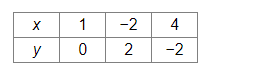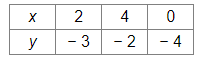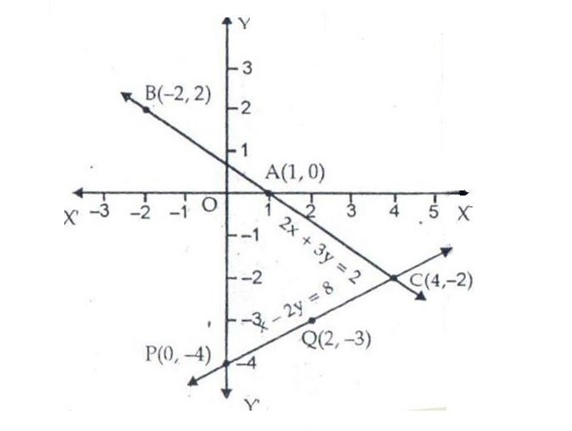# Solve this

Question:

$2 x+3 y=2$

$x-2 y=8$

Solution:

On a graph paper, draw a horizontal line X'OX and a vertical line YOY' representing the x-axis and y-axis, respectively.

2x + 3y = 2
⇒ 3y = (2 − 2x)
⇒ 3y = 2(1 − x)

$\Rightarrow y=\frac{2(1-x)}{3}$  .......(i)

Putting x = 1, we get y = 0
Putting x = − 2, we get y = 2
Putting x = 4, we get y = −2
Thus, we have the following table for the equation 2x + 3y = 2.Now, plot the points A(1, 0), B(− 2 , 2) and C(4, − 2) on the graph paper.
Join AB and AC to get the graph line BC. Extend it on both ways.
Thus, the line BC is the graph of  2x + 3y = 2.

x − 2y = 8
⇒ 2y = x − 8)

$\Rightarrow y=\frac{x-8}{2}$    ......(ii)

Putting x = 2, we get y = −3
Putting x = 4, we get y = −2
Putting x = 0, we get y = −4
Thus, we have the following table for the equation x - 2y = 8.Now, plot the points P(0, − 4) and Q(2, − 3). The point C(4, −2) has already been plotted. Join PQ and QC and extend it on both ways.
Thus, line PC is the graph of x − 2y = 8.The two graph lines intersect at C(4, −2).
∴ x = 4 and y = −2 are the solutions of the given system of equations.